# Fraction Standards 3rd-Grade: Sample Questions

## Unit Fractions and Fraction Strips

3.NF.A.1 - 3.3(C) | Recognize a Unit Fraction

Natalie folded 1 whole fraction strip as pictured: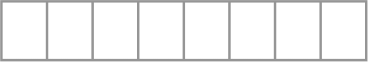What unit fraction is each part equal to?

3.NF.A.1 - 3.3(A) | Recognize a Non-Unit Fraction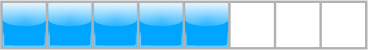What fraction of the strip is shaded?

3.NF.A.1 - 3.3(X) | Understand Numerators and Denominators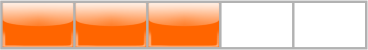What denominator is modeled in this fraction strip?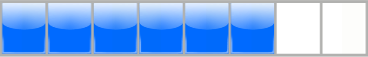What numerator is modeled in this fraction strip?

3.NF.A.1 - 3.3(A) | Model Non-Unit FractionsModel a fraction strip that represents 3/5.

(Partition it into the correct number of piecesand shade the correct # of pieces.)

3.NF.A.1 - 3.3(D) | Compose and Decompose Non-Unit Fractions

Miguel mowed 1/4 of his lawn on Monday.
He mowed another 1/4 on Wednesday
and another 1/4 on Friday.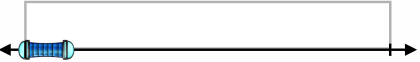Model the fraction of the lawn he mowed altogether.

(Partition and Select.)

3.NF.A.1 - 3.3(E) | Equal shares of identical wholes need not have the same shape

Miguel colors a fraction of identical grids blue and green.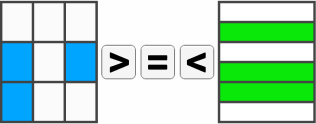Compare the fraction of the grids he colored.

3.NF.A.1 - 3.5(E) | Partitioning an object or set of objects among two or more recipients

Lonny and and his 2 friends win 6
pieces of candy and share them equally.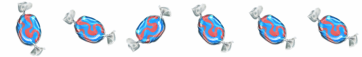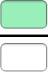What fraction of the candy do each of them get?

## Number Lines

3.NF.A.1 - 3.3(C) | Recognize Unit Fractions on a Number Line

The number line represents a race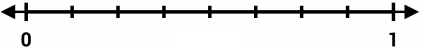What fraction of the race does each unit on the number line represent?

3.NF.A.1 - 3.3(A) | Recognize Non-Unit Fractions on a Number Line

The arrow shows where Elena is in a different race.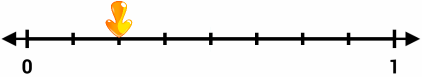What fraction of the race has she completed?

3.NF.A.1 - 3.3(X) | Represent Non-Unit Fractions on a Number Line

Miguel has completed 5/6 of the race.Partition the number line and mark where he is in the race.

## Equivalent Fractions

3.NF.A.1 - 3.3(C) | Recognizing an Equivalent Fraction

Jerry and Frank have identical licorice ropes.
Jerry eats 1/3  of his as shown below: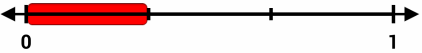Partition the bottom number line
AND select an equivalent fraction.

3.NF.A.1 - 3.3(A) | Creating an Equivalent Fraction

Jerry paints 1/4 of a fence.
Write an equivalent fraction Frank could paint:3.NF.A.1 - 3.3(X) | Explain by using a visual fraction model.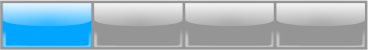by modeling it on the bottom fraction strip.

3.NF.A.1 - 3.3(A) | Whole Number Fraction Equivalency

Jerry has 3 sandwiches.

He cuts each of them into 1/4 pieces.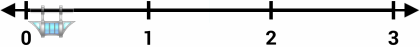Write how many sandwich pieces Jerry has as a fraction.

## Compare Fractions with Same Numerator or Denominator

3.NF.A.1 - 3.3(C) | Compare 2 fractions having the same denominator and numerator.

Compare the following fractions:

(use your toolkit to model them)3.NF.A.1 - 3.3(A) | Compare 2 fractions to solve a problem..

Juan and Karla have identical sandwiches.

Juan partitions his into 6 pieces.

Karla partitions hers into 8 pieces.

They both eat 5 pieces.

Compare how much Juan and Karla ate: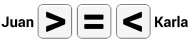Use your toolkit to model it